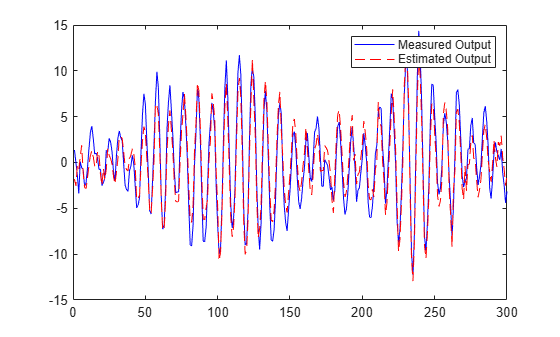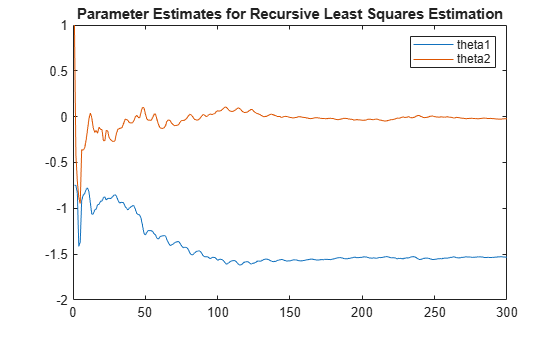# step

Update model parameters and output online using recursive estimation algorithm

## Syntax

``````[EstimatedParameters,EstimatedOutput] = step(obj,y,InputData)``````

## Description

example

``````[EstimatedParameters,EstimatedOutput] = step(obj,y,InputData)``` updates parameters and output of the model specified in System object™, `obj`, using measured output, `y`, and input data.`step` puts the object into a locked state. In a locked state you cannot change any nontunable properties of the object, such as model order, data type, or estimation algorithm.The `EstimatedParameters` and `InputData` depend on the online estimation System object:`recursiveAR` — `step` returns the estimated polynomial A(q) coefficients of a single output AR model using time-series output data. `[A,EstimatedOutput] = step(obj,y)``recursiveARMA` — `step` returns the estimated polynomial A(q) and C(q) coefficients of a single output ARMA model using time-series output data, y. `[A,C,EstimatedOutput] = step(obj,y)``recursiveARX` — `step` returns the estimated polynomial A(q) and B(q) coefficients of a SISO or MISO ARX model using measured input and output data, u and y, respectively. `[A,B,EstimatedOutput] = step(obj,y,u)`.`recursiveARMAX` — `step` returns the estimated polynomial A(q), B(q), and C(q) coefficients of a SISO ARMAX model using measured input and output data, u and y, respectively. `[A,B,C,EstimatedOutput] = step(obj,y,u)`.`recursiveOE` — `step` returns the estimated polynomial B(q), and F(q) coefficients of a SISO Output-Error polynomial model using measured input and output data, u and y, respectively. `[B,F,EstimatedOutput] = step(obj,y,u)`.`recursiveBJ` — `step` returns the estimated polynomial B(q), C(q), D(q), and F(q) coefficients of a SISO Box-Jenkins polynomial model using measured input and output data, u and y, respectively. ```[B,C,D,F,EstimatedOutput] = step(obj,y,u)```.`recursiveLS` — `step` returns the estimated system parameters, θ, of a single output system that is linear in estimated parameters, using regressors H and output data y. `[theta,EstimatedOutput] = step(obj,y,H)`.```

## Examples

collapse all

Create a System object for online parameter estimation of an ARMAX model.

`obj = recursiveARMAX;`

The ARMAX model has a default structure with polynomials of order 1 and initial polynomial coefficient values, `eps`.

Load the estimation data. In this example, use a static data set for illustration.

```load iddata1 z1; output = z1.y; input = z1.u;```

Estimate ARMAX model parameters online using `step`.

```for i = 1:numel(input) [A,B,C,EstimatedOutput] = step(obj,output(i),input(i)); end```

View the current estimated values of polynomial `A` coefficients.

`obj.A`
```ans = 1×2 1.0000 -0.8298 ```

View the current covariance estimate of the parameters.

`obj.ParameterCovariance`
```ans = 3×3 0.0001 0.0001 0.0001 0.0001 0.0032 0.0000 0.0001 0.0000 0.0001 ```

View the current estimated output.

`EstimatedOutput`
```EstimatedOutput = -4.5595 ```

Create a System object for online parameter estimation of an ARMAX model.

`obj = recursiveARMAX;`

The ARMAX model has a default structure with polynomials of order 1 and initial polynomial coefficient values, `eps`.

Load the estimation data. In this example, use a static data set for illustration.

```load iddata1 z1; output = z1.y; input = z1.u; dataSize = numel(input);```

Estimate ARMAX model parameters online using the default recursive estimation algorithm, Forgetting Factor. Change the `ForgettingFactor` property during online parameter estimation.

```for i = 1:dataSize if i == dataSize/2 obj.ForgettingFactor = 0.98; end [A,B,C,EstimatedOutput] = step(obj,output(i),input(i)); end```

The system has two parameters and is represented as:

`$\mathit{y}\left(\mathit{t}\right)={\mathit{a}}_{1}\mathit{u}\left(\mathit{t}\right)+{\mathit{a}}_{2}\mathit{u}\left(\mathit{t}-1\right)$`

Here,

• $\mathit{u}$ and $y$ are the real-time input and output data, respectively.

• $\mathit{u}\left(\mathit{t}\right)$ and $\mathit{u}\left(\mathit{t}-1\right)$ are the regressors, `H`, of the system.

• ${\mathit{a}}_{1}$ and ${\mathit{a}}_{2}$ are the parameters, `theta`, of the system.

Create a System object for online estimation using the recursive least squares algorithm.

`obj = recursiveLS(2);`

Load the estimation data, which for this example is a static data set.

```load iddata3 input = z3.u; output = z3.y;```

Create a variable to store `u(t-1)`. This variable is updated at each time step.

`oldInput = 0;`

Estimate the parameters and output using `step` and input-output data, maintaining the current regressor pair in `H`. Invoke the `step` function implicitly by calling the `obj` system object with input arguments.

```for i = 1:numel(input) H = [input(i) oldInput]; [theta, EstimatedOutput] = obj(output(i),H); estimatedOut(i)= EstimatedOutput; theta_est(i,:) = theta; oldInput = input(i); end```

Plot the measured and estimated output data.

```numSample = 1:numel(input); plot(numSample,output,'b',numSample,estimatedOut,'r--'); legend('Measured Output','Estimated Output');```Plot the parameters.

```plot(numSample,theta_est(:,1),numSample,theta_est(:,2)) title('Parameter Estimates for Recursive Least Squares Estimation') legend("theta1","theta2")```View the final estimates.

`theta_final = theta`
```theta_final = 2×1 -1.5322 -0.0235 ```

## Input Arguments

collapse all

System object for online parameter estimation, created using one of the following commands:

The `step` command updates parameters of the model using the recursive estimation algorithm specified in `obj` and the incoming input-output data.

Output data acquired in real time, specified as a real scalar.

Input data acquired in real time, specified as a scalar or vector of real values depending on the type of System object.

System objectModel Type`InputData`
`recursiveAR`Time-seriesNot Applicable
`recursiveARMA`Time-seriesNot Applicable
`recursiveARX`SISO ARXReal scalar
MISO ARX with N inputsColumn vector of length N, specified as real values
`recursiveARMAX`SISOReal scalar
`recursiveOE`SISOReal scalar
`recursiveBJ`SISOReal scalar
`recursiveLS`Single output system with Np system parametersRegressors, H, specified as a vector of real values of length Np

## Output Arguments

collapse all

Estimated model parameters, returned as vectors of real values. The number of estimated parameters, and so the `step` syntax, depend on the type of System object:

Online Estimation System ObjectEstimated Parameters
`recursiveAR`

Polynomial A(q) coefficients

`recursiveARMA`

Polynomials A(q) and C(q) coefficients

`recursiveARX`

Polynomials A(q) and B(q) coefficients

`recursiveARMAX`

Polynomials A(q), B(q), and C(q) coefficients

`recursiveOE`

Polynomials B(q) and F(q)

`recursiveBJ`

Polynomials B(q), C(q), D(q), and F(q) coefficients

`recursiveLS`

System parameters, θ

Estimated output, returned as a real scalar. The output is estimated using input-output estimation data, current parameter values, and recursive estimation algorithm specified in `obj`.

## Tips

• Starting in R2016b, instead of using the `step` command to update model parameter estimates, you can call the System object with input arguments, as if it were a function. For example, ```[A,EstimatedOutput] = step(obj,y)``` and `[A,EstimatedOutput] = obj(y)` perform equivalent operations.

Introduced in R2015b

## SupportGet trial now